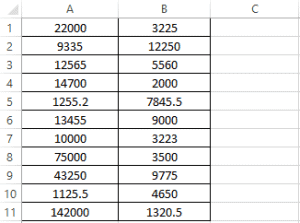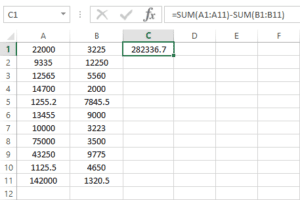## 1. Overview

In this quick tutorial, we’re going to talk about how we can set formulas in a Microsoft Excel spreadsheet using Apache POI through a simple example.

## 2. Apache POI

Apache POI is a popular open-source Java library that provides programmers with APIs to create, modify, and display MS Office files.

It uses Workbook to represent an Excel file and its elements. A Cell in an Excel file can have different types such as FORMULA.

In order to see Apache POI in action, we’re going to set a formula to subtract the sum of the values in columns A and B in an Excel file. The linked file contains the data below:## 3. Dependencies

First, we need to add the POI dependency to our project pom.xml file. To work with Excel 2007+ workbooks, we should use poi-ooxml:

``````<dependency>
<groupId>org.apache.poi</groupId>
<artifactId>poi-ooxml</artifactId>
<version>5.2.0</version>
</dependency>``````

Note that for earlier versions of Excel, we should use the poi dependency instead.

## 4. Cell Lookup

To begin, let’s first open our file and construct the appropriate workbook:

``````FileInputStream inputStream = new FileInputStream(new File(fileLocation));
XSSFWorkbook excel = new XSSFWorkbook(inputStream);``````

Then, we need to create or look up the cell we’re going be using. Using the data shared earlier, we want to edit cell C1.

That’s on the first sheet and the first row, and we can ask POI for the first blank column:

``````XSSFSheet sheet = excel.getSheetAt(0);
int lastCellNum = sheet.getRow(0).getLastCellNum();
XSSFCell formulaCell = sheet.getRow(0).createCell(lastCellNum + 1);``````

## 5. Formulas

Next, we want to set a formula on the cell we’ve looked up.

As stated earlier, let’s subtract the sum of column B from the sum of column A. In Excel, this would be:

``=SUM(A:A)-SUM(B:B)``

And we can write that into our formulaCell with the setCellFormula method:

``formulaCell.setCellFormula("SUM(A:A)-SUM(B:B)");``

Now, this won’t evaluate the formula. To do that, we’ll need to use POI’s XSSFFormulaEvaluator:

``````XSSFFormulaEvaluator formulaEvaluator =
excel.getCreationHelper().createFormulaEvaluator();
formulaEvaluator.evaluateFormulaCell(formulaCell);``````

The result will be set in the first Cell of the next empty column:As we can see, the result is calculated and saved in the first cell of column C. Also the formula is shown in the formula bar.

Note that the FormulaEvaluator class provides us with other methods to evaluate FORMULA in Excel workbooks, like evaluateAll, which will loop over all cells and evaluate them.

## 6. Conclusion

In this tutorial, we showed how to set a formula on a cell in an Excel file in Java using the Apache POI API.

The complete source code for this article is available over on GitHub.

### Get started with Spring and Spring Boot, through the Learn Spring course:

>> CHECK OUT THE COURSE